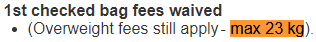# Unit Conversions

## Objective

Solve multi-step word problems that requires metric unit conversions of length, mass, and capacity.

## Common Core Standards

### Core Standards

?

• 4.MD.A.2 — Use the four operations to solve word problems involving distances, intervals of time, liquid volumes, masses of objects, and money, including problems involving simple fractions or decimals, and problems that require expressing measurements given in a larger unit in terms of a smaller unit. Represent measurement quantities using diagrams such as number line diagrams that feature a measurement scale.

?

• 3.MD.A.2

• 4.OA.A.3

## Criteria for Success

?

1. Solve multi-step word problems that requires metric unit conversions of length, mass, and capacity (MP.4).

## Tips for Teachers

?

Let students work on each problem in the Problem Set independently and circulate to see whether students are solving correctly. If not, come back together to discuss how/what to draw on a tape diagram, then allow them to try again on their own.

### Remote Learning Guidance

This lesson does not have any identified priority Anchor Tasks, but students should complete the Problem Set independently rather than skipping the lesson entirely. Find more guidance on adapting our math curriculum for remote learning here.

#### Fishtank Plus

• Problem Set
• Student Handout Editor
• Vocabulary Package

?

Jennifer, Leon, and Veronica see this on their ticket:The weight of their bags is listed below.

 Suitcase Mass Blue 26 kg Black 20 kg 40 g Grey 23,975 g

1. Are each of their bags within the weight limit? If not, which ones exceed it?
2. Can they rearrange their bags so that they are all under the weight limit?
3. If so, how much weight under the limit will they be in total? If not, what might be an object they packed that they could take out of their suitcases to be under the limit?

## Problem Set & Homework

### Discussion of Problem Set

• How did you determine how much apple juice Lisa put in the glass in #2?
• How many total grams of corn did Farmer Asmed sell in #6? How did you solve?

?

### Problem 1

Solve. Show or explain your work.

The average fourth grader is about three times as tall as the average newborn baby. If babies are on average 45 cm 7 mm when they are born, what is the height of the average fourth grader?

### Problem 2

Solve. Show or explain your work.

Jeff is making fruit punch. The recipe includes equal amounts of mango and pineapple juice and 490 mL of orange juice. If the recipe makes 1 L 50 mL, how much of each of the mango and pineapple juices are in the recipe?